# Monge-Ampère equation

A second-order partial differential equation of the form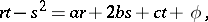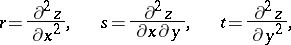with coefficients depending on variables,, the unknown function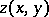, and its first derivatives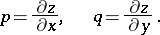The type of a Monge–Ampère equation depends on the sign of the expression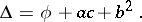If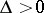, then the Monge–Ampère equation is of elliptic type, ifit is of hyperbolic type and if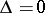it is of parabolic type. A Monge–Ampère equation is invariant under contact transformations (cf. Contact transformation). In particular, the transformationtransforms the equation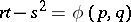into the equation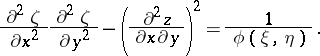The development of the theory of Monge–Ampère equations is mainly connected with the solution of various geometric problems which, when formulated analytically, reduce to the discussion of such equations. For example, the construction of a surface with a given line element reduces to the solution of the Darboux equation, which is a Monge–Ampère equation. In the case of a semi-geodesic parametrization (with line element) this equation takes the form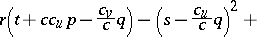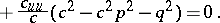The type of the Darboux equation depends on the sign of the Gaussian curvature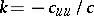. For a hyperbolic Darboux equation (negative Gaussian curvature) the characteristics are asymptotic lines. An application of the Cauchy–Kovalevskaya theorem to Darboux's equation gives a theorem about the existence of a surface with a given line element whose coefficients are analytic functions.

The particularly high level of development of the theory of elliptic Monge–Ampère equations is due to the introduction of the idea of a generalized solution and the application of geometric methods for their solution. In the simplest case of the equation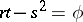, a generalized solution is defined as a convex functionsatisfying the equalitywhereis an arbitrary Borel set in the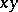-plane in the domain in which the solution is to be discussed, and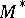is the so-called normal image of, which consists of those points of the-plane for whichandare the angular coefficients of the support planes to the surfaceat points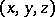projecting into. A regular (twice-differentiable) solution is also a generalized solution. A generalized solution in the case thatis a continuous positive function is smooth; however, the second derivatives need not exist. The construction of a generalized solution essentially reduces to the purely geometric problem of constructing an infinite convex polyhedron with given directions for the finite faces and given functions on these faces. In particular, if the right-hand sideof the equation depends only onand, then this function is the area of the face. Passage to the limit of these polyhedra gives the graph of a generalized solution of the equation. For generalized solutions fairly general existence and uniqueness theorems have been obtained for the solution of the Dirichlet problem. In particular, there is the following theorem. The Dirichlet problem for the equation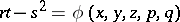in a convex domainis solvable for any continuous boundary values if the curvature of the curve boundingis positive, ifis continuous, positive, non-decreasing in, and, as, has order of growth not exceeding. For a given direction of convexity this solution is unique.

An essential result in the theory of elliptic Monge–Ampère equations is the theorem on regularity of generalized solutions. For the simplest equationthis theorem reads: For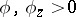every generalized solution with a regular right-hand side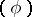is regular. Namely, ifistimes differentiable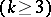, then the generalized solution istimes differentiable. Ifis analytic, then the generalized solution is analytic.

Among the general elliptic Monge–Ampère equations the strongly-elliptic Monge–Ampère equations are the ones most studied. These are the equations for whichand the quadratic form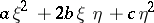is non-negative. The basic results, quoted above for the simplest Monge–Ampère equations, have been extended to the strongly-elliptic case. The idea of a generalized solution has been introduced, and under very general conditions the existence and uniqueness of the solution to the Dirichlet problem as well as regularity of a generalized solution, depending on the regularity of the coefficients of the equation, has been proved. Darboux's equation for a line element with positive curvature is, in general, not strongly elliptic. A generalized solution for it is defined as the-coordinate of a surface realizing the given line element. Darboux's equation has a generalized solution in any domain that is convex in the sense of the given metric (the geodesic curvature of the boundary should be positive). In any such domain, under sufficiently general conditions, the solvability of the Dirichlet problem has been proved. A generalized solution is regular if the coefficients of the line element are regular. The generalized solution is analytic if the coefficients are analytic.

Important results have been obtained for elliptic Monge–Ampère equations on manifolds homeomorphic to the sphere. In particular, two classical problems reduce to such equations: the Weyl problem and the Minkowski problem. The solutions of these problems, obtained by passage to the limit over polyhedra, are generalized. Regularity of these solutions is obtained from theorems on the regularity of generalized solutions.

Monge–Ampère equations were considered by G. Monge (1784) and A. Ampère (1820).

How to Cite This Entry:
Monge-Ampère equation. Encyclopedia of Mathematics. URL: http://encyclopediaofmath.org/index.php?title=Monge-Amp%C3%A8re_equation&oldid=24508
This article was adapted from an original article by A.V. Pogorelov (originator), which appeared in Encyclopedia of Mathematics - ISBN 1402006098. See original article